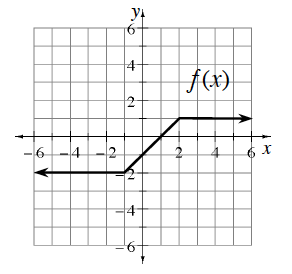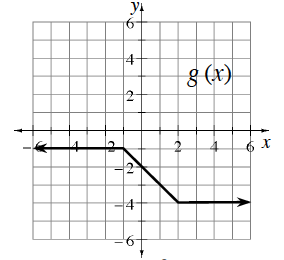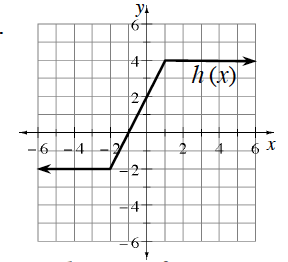### Home > PC3 > Chapter 4 > Lesson 4.2.1 > Problem4-60

4-60.

The graph of $y=f(x)$ is shown at right. Below are graphs of $y=g(x)$ and $y=h(x)$, which are transformations of $y=f(x)$. Write equations for $g(x)$ and $h(x)$ in terms of $f(x)$.1.This curve has been vertically reflected.
A vertical reflection of $y=f(x)$ results in a ray of $y=−1$ on the right side. How does that compare to this curve?

1.This curve has been vertically stretched.
The "bounds" of $y=f(x)$ are $y=−2$ and $y=1$, so think of the "height" as $3$ units.
How does this compare to the "height" of $y=g(x)$?

If $y=f(x)$ is vertically stretched by a factor of $2$, the point where the curve bends, $(2,1)$ will end up at $(2,2)$. Where is this point on $y=h(x)$?